# Cartan subgroup

(diff) ← Older revision | Latest revision (diff) | Newer revision → (diff)

of a groupA maximal nilpotent subgroup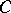ofeach normal subgroup of finite index of which has finite index in its normalizer in. Ifis a connected linear algebraic group over a field of characteristic zero, then a Cartan subgroup ofcan also be defined as a closed connected subgroup whose Lie algebra is a Cartan subalgebra of the Lie algebra of. An example of a Cartan subgroup is the subgroupof all diagonal matrices in the group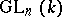of all non-singular matrices.

In a connected linear algebraic group, a Cartan subgroup can also be defined as the centralizer of a maximal torus of, or as a connected closed nilpotent subgroupwhich coincides with the connected component of the identity (the identity component) of its normalizer in. The sets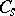andof all semi-simple and unipotent elements of(see Jordan decomposition) are closed subgroups in, and. In addition,is the unique maximal torus oflying in. The dimension of a Cartan subgroup ofis called the rank of. The union of all Cartan subgroups ofcontains an open subset ofwith respect to the Zariski topology (but is not, in general, the whole of). Every semi-simple element oflies in at least one Cartan subgroup, and every regular element in precisely one Cartan subgroup. Ifis a surjective morphism of linear algebraic groups, then the Cartan subgroups of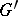are images with respect toof Cartan subgroups of. Any two Cartan subgroups ofare conjugate. A Cartan subgroup of a connected semi-simple (or, more generally, reductive) groupis a maximal torus in.

Let the groupbe defined over a field. Then there exists ina Cartan subgroup which is also defined over; in fact,is generated by its Cartan subgroups defined over. Two Cartan subgroups ofdefined overneed not be conjugate over(but in the case whenis a solvable group, they are conjugate). The variety of Cartan subgroups ofis rational over.

Letbe a connected real Lie group with Lie algebra. Then the Cartan subgroups ofare closed in(but not necessarily connected) and their Lie algebras are Cartan subalgebras of. Ifis an analytic subgroup in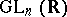and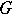is the smallest algebraic subgroup ofcontaining, then the Cartan subgroups ofare intersections ofwith the Cartan subgroups of. In the case whenis compact, the Cartan subgroups are connected, Abelian (being maximal tori) and conjugate to one another, and every element oflies in some Cartan subgroup.

How to Cite This Entry:
Cartan subgroup. Encyclopedia of Mathematics. URL: http://encyclopediaofmath.org/index.php?title=Cartan_subgroup&oldid=11216
This article was adapted from an original article by V.L. Popov (originator), which appeared in Encyclopedia of Mathematics - ISBN 1402006098. See original article